• 1、在一张图上画多条曲线 import matplotlib.pyplot as...x = np.linspace(-3,3,50) #设置横轴的取值点 y1 = 2 * x + 1 #曲线1 y2 = x**2 #曲线2 plt.figure(num=3,figsize=(8,5)) plt.plot(x,y2) plt.plot(x,y1,...
1、在一张图上画多条曲线

import matplotlib.pyplot as plt
import numpy as np

x = np.linspace(-3,3,50)  #设置横轴的取值点

y1 = 2 * x + 1
#曲线1
y2 = x**2
#曲线2
plt.figure(num=3,figsize=(8,5))
plt.plot(x,y2)
plt.plot(x,y1,color='red',linewidth=1,linestyle='--')

plt.show()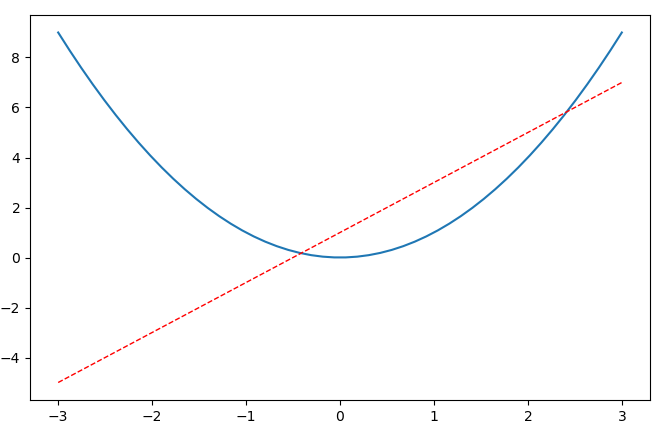2、设置坐标轴的长度

plt.figure(num=3,figsize=(8,5))

3、设置坐标轴的取值范围

import matplotlib.pyplot as plt
import numpy as np

x = np.linspace(-3,3,50)

y1 = 2 * x + 1
y2 = x**2

# plt.figure(num=1)
# plt.plot(x,y1)

plt.figure(num=3,figsize=(8,5))
plt.plot(x,y2)
plt.plot(x,y1,color='red',linewidth=1,linestyle='--')
plt.xlim((-1,2))
plt.ylim((-1,2))

4、设置坐标轴的名称

import matplotlib.pyplot as plt
import numpy as np

x = np.linspace(-3,3,50)

y1 = 2 * x + 1
y2 = x**2

# plt.figure(num=1)
# plt.plot(x,y1)

plt.figure(num=3,figsize=(8,5))
plt.plot(x,y2)
plt.plot(x,y1,color='red',linewidth=1,linestyle='--')
plt.xlim((-1,2))
plt.ylim((-1,2))
plt.xlabel('XXX')
plt.ylabel('YYY')

plt.show()

5、重新设置坐标轴上的刻度

import matplotlib.pyplot as plt
import numpy as np

x = np.linspace(-3,3,50)

y1 = 2 * x + 1
y2 = x**2

# plt.figure(num=1)
# plt.plot(x,y1)

plt.figure(num=3,figsize=(8,5))
plt.plot(x,y2)
plt.plot(x,y1,color='red',linewidth=1,linestyle='--')
plt.xlim((-1,2))
plt.ylim((-1,2))
plt.xlabel('XXX')
plt.ylabel('YYY')
#
new_ticks = np.linspace(-1,2,10)
print(new_ticks)
plt.xticks(new_ticks)

plt.show()


展开全文• 今天用gnuplot画图，发现自动设置set xlabel 的话，坐标名称位置不是那么好看，想要自己调整一下，似乎没找到专门改坐标轴名字位置的办法。 但是有一个可以灵活设置label的方法： set label "the name of your ...
今天用gnuplot画图，发现自动设置set xlabel 的话，坐标名称位置不是那么好看，想要自己调整一下，似乎没找到专门改坐标轴名字位置的办法。
但是有一个可以灵活设置label的方法：
set label "the name of your label"  0,0
或者pm3d中：
set label "the name of your label"  0,0,0
三维时候如果不写全三个坐标位置的话，就会变成二维图了。

还有一个，设置网格线，
set grid [n]
后面的n是可选的，如果写数字的话，可以改变不同的网格线颜色，默认是0，虚线。
这几天还要常常画图，发现gnuplot是相当强大了，而且又简单易用。真好，哈哈

展开全文• ## matplotlib设置坐标轴

万次阅读 多人点赞 2017-05-04 15:59:30
在使用matplotlib模块时画坐标图时，往往需要对坐标轴设置很多参数，这些参数包括横纵坐标轴范围、坐标轴刻度大小、坐标轴名称等 在matplotlib中包含了很多函数，用来对这些参数进行设置。plt.xlim、plt.ylim 设置...
在使用matplotlib模块时画坐标图时，往往需要对坐标轴设置很多参数，这些参数包括横纵坐标轴范围、坐标轴刻度大小、坐标轴名称等
在matplotlib中包含了很多函数，用来对这些参数进行设置。

plt.xlim、plt.ylim                            设置横纵坐标轴范围
plt.xlabel、plt.ylabel        设置坐标轴名称
plt.xticks、plt.yticks设置坐标轴刻度

以上plt表示matplotlib.pyplot

例子


#导入包
import matplotlib.pyplot as plt
import numpy as np

#创建数据
x = np.linspace(-5, 5, 100)
y1 = np.sin(x)
y2 = np.cos(x)

#创建figure窗口
plt.figure(num=3, figsize=(8, 5))
#画曲线1
plt.plot(x, y1)
#画曲线2
plt.plot(x, y2, color='blue', linewidth=5.0, linestyle='--')
#设置坐标轴范围
plt.xlim((-5, 5))
plt.ylim((-2, 2))
#设置坐标轴名称
plt.xlabel('xxxxxxxxxxx')
plt.ylabel('yyyyyyyyyyy')
#设置坐标轴刻度
my_x_ticks = np.arange(-5, 5, 0.5)
my_y_ticks = np.arange(-2, 2, 0.3)
plt.xticks(my_x_ticks)
plt.yticks(my_y_ticks)

#显示出所有设置
plt.show()

结果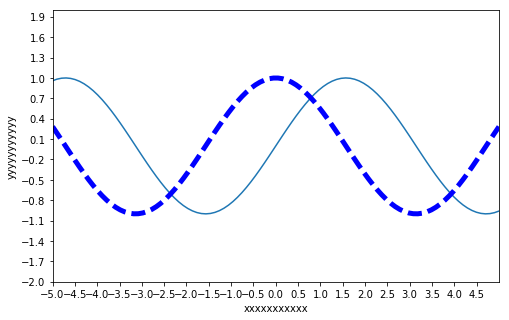展开全文函数
• 在使用matplotlib模块时画坐标图时，往往需要对坐标轴设置很多参数，这些参数包括横纵坐标轴范围、坐标轴刻度大小、坐标轴名称等 xlim():设置x坐标轴范围 ylim():设置y坐标轴范围 xlabel():设置x坐标轴名称 ylabel...
在使用matplotlib模块时画坐标图时，往往需要对坐标轴设置很多参数，这些参数包括横纵坐标轴范围、坐标轴刻度大小、坐标轴名称等

xlim():设置x坐标轴范围
ylim():设置y坐标轴范围
xlabel():设置x坐标轴名称
ylabel():设置y坐标轴名称
xticks():设置x轴刻度
yticks():设置y轴刻度

#创建数据
x = np.linspace(-5, 5, 100)
y1 = np.sin(x)
y2 = np.cos(x)

#创建figure窗口，figsize设置窗口的大小
plt.figure(num=3, figsize=(8, 5))
#画曲线1
plt.plot(x, y1)
#画曲线2
plt.plot(x, y2, color='blue', linewidth=5.0, linestyle='--')
#设置坐标轴范围
plt.xlim((-5, 5))
plt.ylim((-2, 2))
#设置坐标轴名称
plt.xlabel('xxxxxxxxxxx')
plt.ylabel('yyyyyyyyyyy')
#设置坐标轴刻度
my_x_ticks = np.arange(-5, 5, 0.5)
#对比范围和名称的区别
#my_x_ticks = np.arange(-5, 2, 0.5)
my_y_ticks = np.arange(-2, 2, 0.3)
plt.xticks(my_x_ticks)
plt.yticks(my_y_ticks)

#显示出所有设置
plt.show()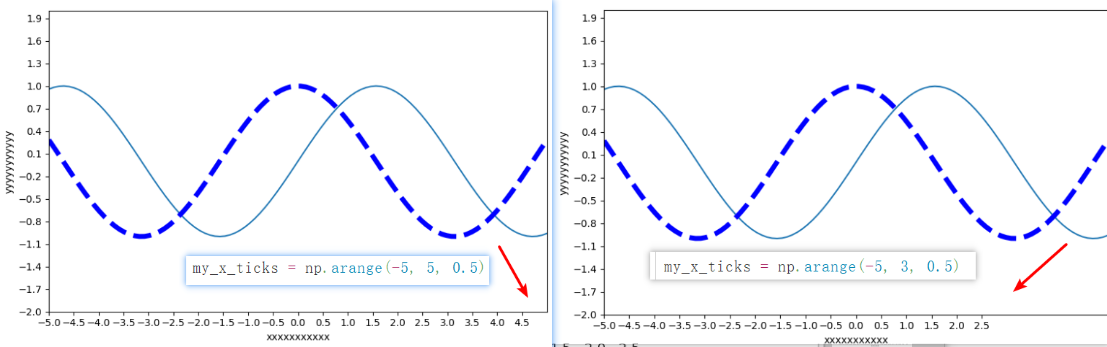import matplotlib.pyplot as plt
import numpy as np

x = np.linspace(-3, 3, 50)
y1 = 2*x + 1
y2 = x**2

plt.figure()
plt.plot(x, y2)
plt.plot(x, y1, color='red', linewidth=1.0, linestyle='--')

plt.xlim((-1, 2))
plt.ylim((-2, 3))
plt.xlabel('I am x')
plt.ylabel('I am y')
plt.show()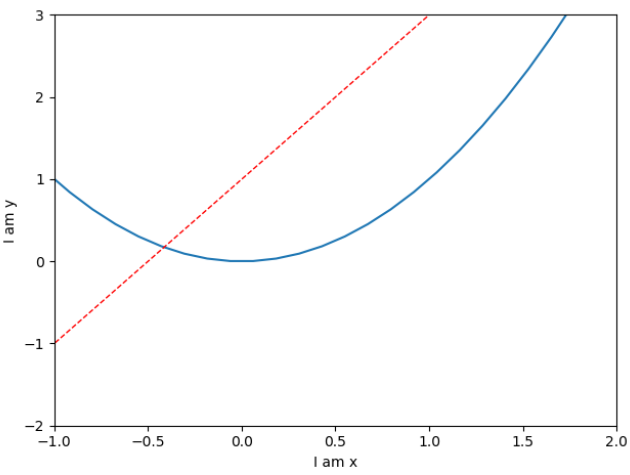x = np.linspace(-3, 3, 50)
y1 = 2*x + 1
y2 = x**2

plt.figure()
plt.plot(x, y2)
plt.plot(x, y1, color='red', linewidth=1.0, linestyle='--')

plt.xlim((-1, 2))
plt.ylim((-2, 3))
plt.xlabel('I am x')
plt.ylabel('I am y')

new_ticks = np.linspace(-1, 2, 5)
print(new_ticks)
plt.xticks(new_ticks)

plt.yticks([-2, -1.8, -1, 1.22, 3],[r'$really\ bad$', r'$bad$', r'$normal$', r'$good$', r'$really\ good$'])
plt.show()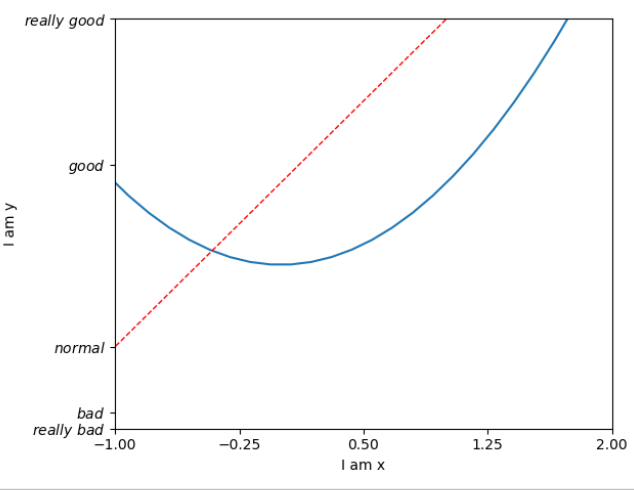设置坐标轴–边框

gca():获取当前坐标轴信息
.spines:设置边框
.set_color:设置边框颜色：默认白色
.spines:设置边框
.xaxis.set_ticks_position:设置x坐标刻度数字或名称的位置
.yaxis.set_ticks_position:设置y坐标刻度数字或名称的位置
.set_position:设置边框位置


x = np.linspace(-3, 3, 50)
y1 = 2*x + 1
y2 = x**2

plt.figure()
plt.plot(x, y2)
plt.plot(x, y1, color='red', linewidth=1.0, linestyle='--')
plt.xlim((-1, 2))
plt.ylim((-2, 3))

new_ticks = np.linspace(-1, 2, 5)
plt.xticks(new_ticks)
plt.yticks([-2, -1.8, -1, 1.22, 3],['$really\ bad$', '$bad$', '$normal$', '$good$', '$really\ good$'])

ax = plt.gca()
#设置上边和右边无边框
ax.spines['right'].set_color('none')
ax.spines['top'].set_color('none')
plt.show()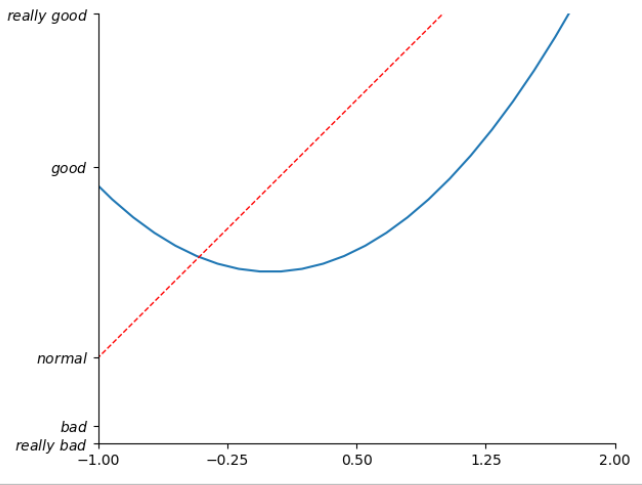调整坐标轴:
ax = plt.gca()
#设置上边和右边无边框
ax.spines['right'].set_color('none')
ax.spines['top'].set_color('none')
#设置x坐标刻度数字或名称的位置
ax.xaxis.set_ticks_position('bottom')
#设置边框位置
ax.spines['bottom'].set_position(('data', 0))

plt.show()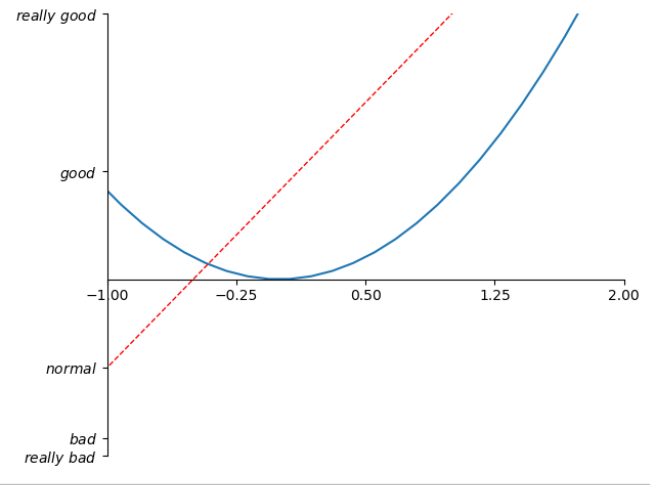ax = plt.gca()
#设置上边和右边无边框
ax.spines['right'].set_color('none')
ax.spines['top'].set_color('none')
#设置x坐标刻度数字或名称的位置
ax.xaxis.set_ticks_position('bottom')
#设置边框位置
ax.spines['bottom'].set_position(('data', 0))
ax.yaxis.set_ticks_position('left')
ax.spines['left'].set_position(('data',0))

plt.show()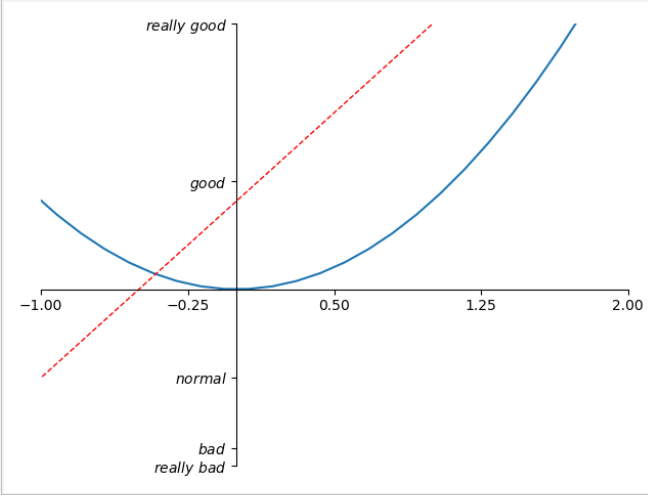matplotlib.pyplot.text(x, y, s, fontdict=None, withdash=False, **kwargs)
x, y：表示坐标；
s：字符串文本；
fontdict：字典，可选；
kw：
fontsize=12,
horizontalalignment=‘center’、ha=’cener’
verticalalignment=’center’、va=’center’

#!/usr/bin/python
#coding: utf-8

import numpy as np
import matplotlib.pyplot as plt

x = np.arange(-10, 11, 1)  #形成一个数组，第三个参数表示步长，#start,end,step
y = x ** 2

plt.plot(x, y)

# 第一个参数是x轴坐标
# 第二个参数是y轴坐标
# 第三个参数是要显式的内容
# alpha 设置字体的透明度
# family 设置字体
# size 设置字体的大小
# style 设置字体的风格
# wight 字体的粗细
# bbox 给字体添加框，alpha 设置框体的透明度， facecolor 设置框体的颜色
plt.text(-3, 20, "function: y = x * x", size = 15, alpha = 0.2)
plt.text(-3, 40, "function: y = x * x", size = 15,\
family = "fantasy", color = "r", style = "italic", weight = "light",\
bbox = dict(facecolor = "r", alpha = 0.2))

plt.show()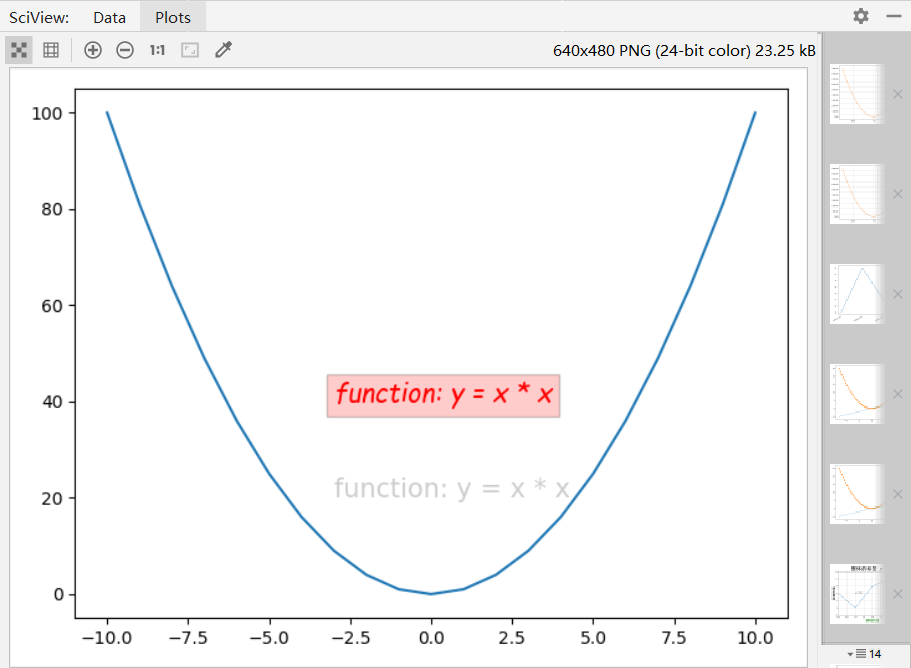添加数据标签
# -*- coding: utf-8 -*-
import time
import matplotlib.pyplot as plt

def showResult(xList, yList, title, xLabel, yLabel):
plt.plot(xList, yList, 'g*-')
plt.title(title)
plt.xlabel(xLabel)
plt.ylabel(yLabel)
for x, y in zip(xList, yList):
plt.text(x, y+0.3, str(y), ha='center', va='bottom', fontsize=10.5)
plt.savefig('fig'+str(int(time.time()))+'.jpg')
plt.show()

x_arr = [1, 2, 3, 4, 5, 6]
y_arr = [1, 4, 9, 16, 25, 36]
showResult(x_arr, y_arr, 'title', 'x', 'y')


其中
for x, y in zip(xList, yList):
plt.text(x, y+0.3, '%.0f'%y, ha='center', va='bottom', fontsize=10.5)


逐个获取需要标注的点的横纵坐标 x与 y，然后在位置 (x, y+0.3) 处以 10.5 的字体显示出 y 的值，‘center’ 和 ‘bottom’ 分别指水平和垂直方向上的对齐方式。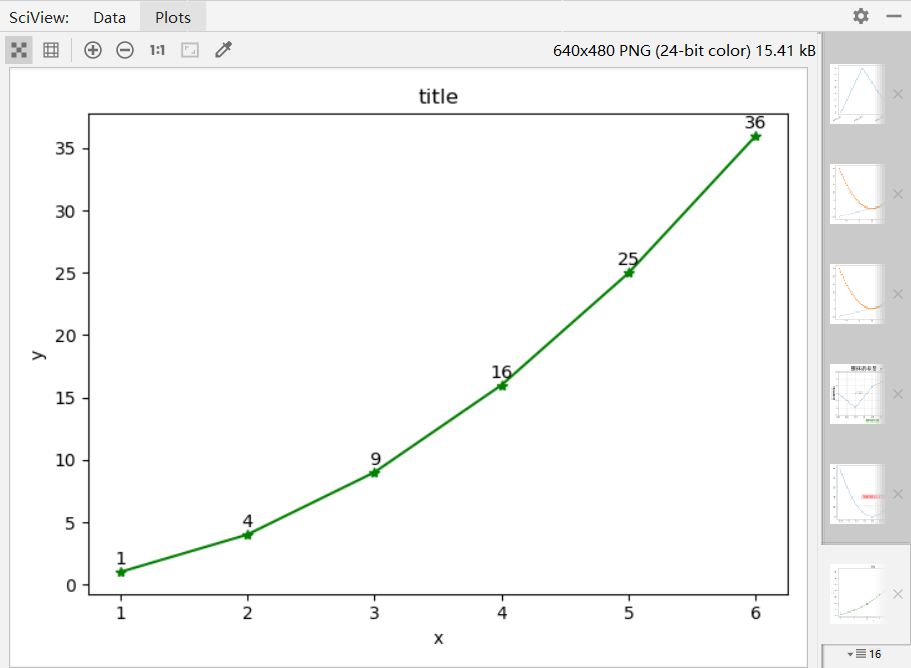展开全文• ## R语言作图：坐标轴设置

万次阅读 多人点赞 2018-02-04 20:51:24
要绘制一张赏心悦目的统计图表，坐标轴设置至关重要。在R语言底层作图中，对坐标轴的调整主要通过调整plot函数、axis函数和title函数的一系列参数完成。 plot(x,y, ...) axis(side,at = NULL, labels = TRUE, ...R 可视化
• 1.去除横纵坐标值 plt.xticks([]) # 去掉横坐标值 plt.yticks([]) # 去掉纵坐标值 2.设置横纵坐标名称 ax1.set_xlabel('Output class',fontsize=24) #...3.设置坐标轴刻度大小 plt.tick_params(labelsize=14) ...python
• 在使用matplotlib模块时画坐标图时，往往需要对坐标轴设置很多参数，这些参数包括横纵坐标轴范围、坐标轴刻度大小、坐标轴名称等  在matplotlib中包含了很多函数，用来对这些参数进行设置。 plt.xlim、plt.ylim ...
• 在使用matplotlib模块时画坐标图时，往往需要对坐标轴设置很多参数，这些参数包括横纵坐标轴范围、坐标轴刻度大小、坐标轴名称等 在matplotlib中包含了很多函数，用来对这些参数进行设置。 plt.xlim、plt.ylim ...python matplotlib plt
• 可以用matlab提供的坐标轴设置函数axis进行设置（对当前坐标轴进行设置），具体用法如下： plot(x, y); // 画图后用axis函数设置坐标轴的范围 axis([xmin xmax ymin ymax]); % 设置坐标轴在指...
• 旋转： nameRotate, 旋转度数 名称与坐标轴间距: nameGap , 间距 大体位置调整：nameLocation, end、start、center三种...but想要是坐标轴名称放到任意位置的话，比如： 可以使用 nameTextStyle.padding来做调整 ...echarts
• 在利用matplotlib绘图时，常常需要添加图例与坐标轴名称，而在论文中，对中英文有严格的不同的字体要求，但默认的方法中(如下所示)，无法分别为中文、英文指定不同字体(如下图)，因此需要借助其他方法。 # -*- co.....python matplotlib
• 但是我们的心中想要达到的目标图为下图，那么就需要对坐标轴进行设置。 解决方案 这里要介绍matplotlib绘图辅助工具包mpl_toolkits，里面包含了一系列对于坐标轴设置的方法。 首先导入辅助工具包里面的轴系设置包 ...Python 程序员
• 同一个坐标轴上的两个字母 X 和 m， 希望X是斜体，m是正体。 figure,xlabel('\itX, m')%显示x坐标 % 显示结果： X 和 M 都变成斜体了。 在MATLAB中，该如何实现X是斜体，m是正体。 解决以上问题： figure,...
• 坐标轴名称左右偏移 xAxis : [ { name:'\n\n\n23:00',//换行调整高度 nameLocation:'end', /*坐标轴名字向左偏移begin*/ nameTextStyle: { fontSize:12, padding: [0, 0, 0, -30] }, /*坐echarts
• 在上周的文章当中我们介绍了如何通过xlabel和ylabel设置坐标轴名称，以及这两个函数的花式设置方法，可以设置出各种各样的名称显示方法。今天我们来介绍介绍其他的设置。 xlim、ylim 我们首先来介绍坐标轴的范围，...python 大数据
• 使用plt.xlabel设置x坐标轴名称：’I am x’； 使用plt.ylabel设置y坐标轴名称：’I am y’； plt.xlim((-1, 2)) plt.ylim((-2, 3)) plt.xlabel('I am x') plt.ylabel('I am y') plt.show() 使用np.linspace定义...
• xAxes: [{  scaleLabel: {  display: true,  labelString: '本月日期'  }, ...坐标轴名称即如下设置： scaleLabel: {  display: true,  labelString: '本月日期'  },js chart.js
• - 多子图的画法 - 设置坐标范围 - 设置坐标的显示间隔 - 设置figure的大标题 - 设置x和y名称
• Sub 批量改图表坐标轴名称() Dim co As ChartObject i = 0 For Each co In ActiveSheet.ChartObjects '处理当前工作表中所有的图表 i = i + 1 co.Chart.Axes(xlCategory).HasTitle = True '显示横轴标题 co....
• XY坐标轴范围及名称 import numpy as np import matplotlib.pyplot as plt x = np.linspace(-3, 3, 50) #X axis data y1 = np.sin(x)*x**2 +1 plt.figure() plt.plot(x, y1) plt.scatter(x, y1, c='r')# set colormatplotlib
• matplotlib, .set_title(), .set_xlabel(), .set_ylabel(), 添加图名和坐标轴名称
• 2.x轴坐标向右 ActiveChart.ChartArea.Select ActiveChart.Axes(xlCategory).Select Seletion.MajorTickMark = xlInside   3.Y轴坐标向上 Selection.MajortickMark = xlInside 转载于:...
• 如何对图例（legend）和坐标轴（xlabel、ylabel）字体大小的调节。matlab
• 文章目录type_ : 坐标轴类型name : 坐标轴名称name_textstyle_opts : 坐标轴名称的文字样式name_gap ：坐标轴名称与轴线之间的距离name_rotate ：坐标轴名字旋转，角度值name_location：坐标轴名称显示位置ispython 数据分析 数据可视化
• 修改标题、坐标轴刻度、坐标轴名称的大小，用到了cex.axis、cex.lab、cex.main函数，其中，cex.axis表示修改坐标轴刻度字体大小，cex.lab表示修改坐标轴名称字体大小，cex.main表示修改标题字体大小 如代码所示： ...
• 在使用matplotlib模块时画坐标图时，往往需要对坐标轴设置很多参数，这些参数包括横纵坐标轴范围、坐标轴刻度大小、坐标轴名称等 在matplotlib中包含了很多函数，用来对这些参数进行设置。 我们可以对坐标轴进行设置......